## Simulink functions: Simulink Function block, exported Stateflow graphical and MATLAB functions

Simulink® functions have an interface with input and output arguments similar to programming languages. You can create the function definition for a Simulink function using:

• Stateflow® state transitions in a graphical function exported from a Stateflow chart.

• MATLAB® code in a MATLAB function exported from a Stateflow chart.

The following sections show how to create a Simulink function for the function ```y = timestwo(x)```. The function multiplies a value (`x`) from a caller by `2`, and then sends the calculated value (`y`) back to the caller. To call the functions, see Simulink function callers: Function Caller block, MATLAB Function block, Stateflow chart.

Set up a Simulink Function block to receive data through an input argument from a function caller, and then pass a calculated value back through an output argument.

2. On the block face, enter the function prototype .

`y = timestwo(x)`3. Double-click the block to open the subsystem defining the function algorithm.

4. Add a Gain block and set the Gain parameter to `2`.### Create a Simulink function using an exported graphical function from a Stateflow chart

Set up a graphical function in a Stateflow chart to receive data through an input argument from a function caller and pass the calculated value back through an output argument. Set chart parameters to export the function to a Simulink model.

#### Define a graphical function In a Stateflow chart

Create a graphical function in a Stateflow chart. Define the function interface and function definition.

1. Add a Stateflow Chart to your Simulink model. Double-click on the Simulink block diagram. In the search box, enter `chart`, and then from the search results, select `Chart`.

2. Double-click to open the chart.

3. Add a graphical function. From the left-side toolbar, click and drag the graphical function icon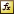onto the chart.

4. Define the function interface. In the function box, replace the `?` with the function interface ```y = timestwo(x)```.

5. Define the function algorithm. Click the transition arrow and replace the `?` with`{y = 2*x}`.Test the graphical function.  Test the graphical function within the Stateflow chart before exporting to a Simulink model.

1. Add a default transition in the chart for testing the function. From the left-side toolbar, click and drag a default transition arrow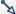onto the chart.

2. Double-click the arrow and replace the `?` with ```{y1 = timestwo(x1)}```.

3. Add an input port to the chart. Open the Model Explorer. In the left pane, select `Chart`. From the menu, select Add > Data. Set Name to `x1` and Scope to `Input`.

4. Add an output port to the chart. From the menu, select Add > Data. Set Name to `y1` and Scope to `Output`.

5. Add a Sine Wave block to provide test data for the input and a Scope block to view results from the output..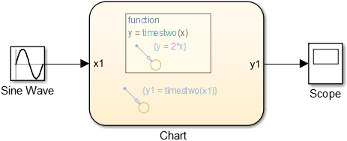6. Run a simulation.

#### Set argument parameters for a graphical function

Specify the size, complexity, and type of the function input and output arguments. A chart can export only functions with fully specified prototypes.

1. Open the Model Explorer. On the Modeling tab and from the Design section, select Model Explorer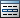.

2. In the left pane, select the graphical function.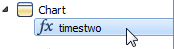3. From the Column View list in the middle pane, select `Stateflow`. Select the filter icon, and then from the toggle list, select All Stateflow Objects. From the center pane table, select an input or output argument.4. In the right pane, set Size to 1 (scaler), Set Complexity to `Off` (real number), and set Type to `double`.5. Repeat steps `2` and `3` with the output function argument `y`.

#### Set export function parameters for a graphical function

Set parameters to export a graphical function to a Simulink model from a Stateflow chart during a simulation.

1. Open the Model Explorer.

2. In the left pane, select the chart containing the graphical function.3. In the property dialog box on the right side, select the Export Chart Level Functions check box, click the button, and then select the Treat Exported Functions as Globally Visible check box.

If you are calling the exported graphical function from another Stateflow chart (not the chart that exported the graphical function), you do not need to select the Treat Exported Functions as Globally Visible check box.

### Create a Simulink function using an exported MATLAB function from a Stateflow chart

Set up a MATLAB function in a Stateflow chart to receive data through an input argument from a function caller and then pass a calculated value back through an output argument. Set chart parameters to export the function to a Simulink model.

#### Define a MATLAB function in a Stateflow chart

Create a MATLAB function in a Stateflow chart. Define the function interface and function definition.

1. Add a Stateflow Chart to your Simulink model. Double-click on the block diagram. In the search box, enter `chart`, and then from the search results, select `Chart`.

2. Open the chart.

3. Add a MATALB function. From the left-side toolbar, click and drag the graphical function icononto the chart.

4. Define the function interface. In the function box, replace the `?` with the function interface ```y = timestwo(x)```.5. Double-click the function box to open the MATLAB code editor. Define the function algorithm with the MATLAB code.Test the Matlab function.  Test the MATLAB function within a Stateflow chart before exporting to a Simulink model.

1. Add a default transition in the chart for testing the function. From the left-side toolbar, click and drag a default transition arrowonto the chart.

2. Double-click the arrow and replace the `?` with ```{y1 = timestwo(x1)}```.

3. Add an input port to the chart. Open the Model Explorer. In the left pane, select `Chart`. From the menu, select Add > Data. Set Name to `x1` and Scope to `Input`.

4. Add an output port to the chart. From the menu, select Add > Data. Set Name to `y1` and Scope to `Output`.

5. Add a Sine Wave block to provide test data for the input and a Scope block to view results from the output..6. Run a simulation.

#### Set the argument parameters for a MATLAB function

Specify the size, complexity, and type of the function input and output arguments. A chart can export only functions with fully specified prototypes.

1. Open the Model Explorer. On the Modeling tab and from the Design section, select Model Explorer.

2. In the left pane, select the MATLAB function.3. From the Column View list in the middle pane, select `Stateflow`. Select the filter icon, and then from the toggle list, select All Stateflow Objects. From the center pane table, select an input or output argument.4. In the right pane, set Size to 1 (scaler), Set Complexity to `Off` (real number), and set Type to `double`.5. Repeat steps `2` and `3` with the output function argument `y`.

#### Set export function parameters for a MATLAB function

Set parameters to export a MATLAB function from a Stateflow chart during a simulation.

1. Open the Model Explorer.

2. From the left pane, select the chart containing the MATLAB function.3. In the property dialog box on the right side, select the Export Chart Level Functions check box, click the button, and then select the Treat Exported Functions as Globally Visible check box.

If you are calling the exported MATLAB function from another Stateflow chart (not the chart that exported the MATLAB function), you do not need to select the Treat Exported Functions as Globally Visible check box.